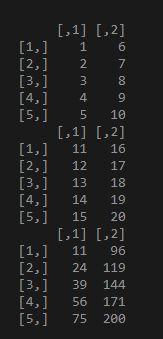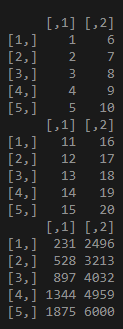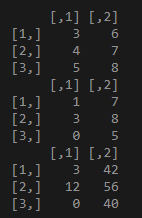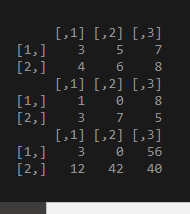# Elementwise Matrix Multiplication in R

• Last Updated : 26 Mar, 2021

In a matrix, as we know rows are the ones that run horizontally and columns are the ones that run vertically. In this article, we are going to perform element-wise matrix multiplication in R programming.

Approach

• Create a matrix
• Multiply two matrices
• Verify the result.

Element-wise multiplication using “*” operator:

Syntax: matrix1*matrix*2…..matrix n

Example 1:

This code shows the element-wise multiplication of two matrices data1 and data2, Data comprises 5 rows and 2 columns:

## R

 `# Creating matrices 10 elements each using``# range operator ":"``data1 <- ``matrix``(1:10, nrow = 5) ``print``(data1)`` ` `data2 <- ``matrix``(11:20, nrow = 5) ``print``(data2)`` ` `# perform element wise multiplication``print``(data1*data2)`

Output:Example 2:

This code for multiplication of multiple matrices data1,data2,data3. All data comprises 5 rows created using the range operator.

## R

 `# Creating matrices 10 elements each ``# using range operator ":"``data1 <- ``matrix``(1:10, nrow = 5) ``print``(data1)`` ` `data2 <- ``matrix``(11:20, nrow = 5) ``print``(data2)`` ` `data3 <- ``matrix``(21:30, nrow = 5)`` ` `# perform element wise multiplication``print``(data1*data2*data3)`

Output:Example 3:

This code shows the matrix is created using vectors. And matrix multiplication is done.

## R

 `# vector a``a = ``c``(3, 4, 5, 6, 7, 8)`` ` `# vector b``b=``c``(1, 3, 0, 7, 8, 5)`` ` `# Creating matrices using vector``data1 <- ``matrix``(a, nrow = 3) ``print``(data1)`` ` `data2 <- ``matrix``(b, nrow = 3) ``print``(data2)`` ` `print``(data1*data2)`

Output:Example 4:

An example that shows multiplication column arrangement and matrices data1 and data2 and multiplied. Column wise we are going to perform matrix multiplication data1 and data2 comprises 3 columns and elements are created using vector.

## R

 `# vector a``a = ``c``(3, 4, 5, 6, 7, 8)`` ` `# vector b``b = ``c``(1, 3, 0, 7, 8, 5)`` ` `# Creating matrices using vector``data1 <- ``matrix``(a, ncol = 3) ``print``(data1)`` ` `data2 <- ``matrix``(b, ncol = 3) ``print``(data2)`` ` `print``(data1*data2)`

Output:My Personal Notes arrow_drop_up